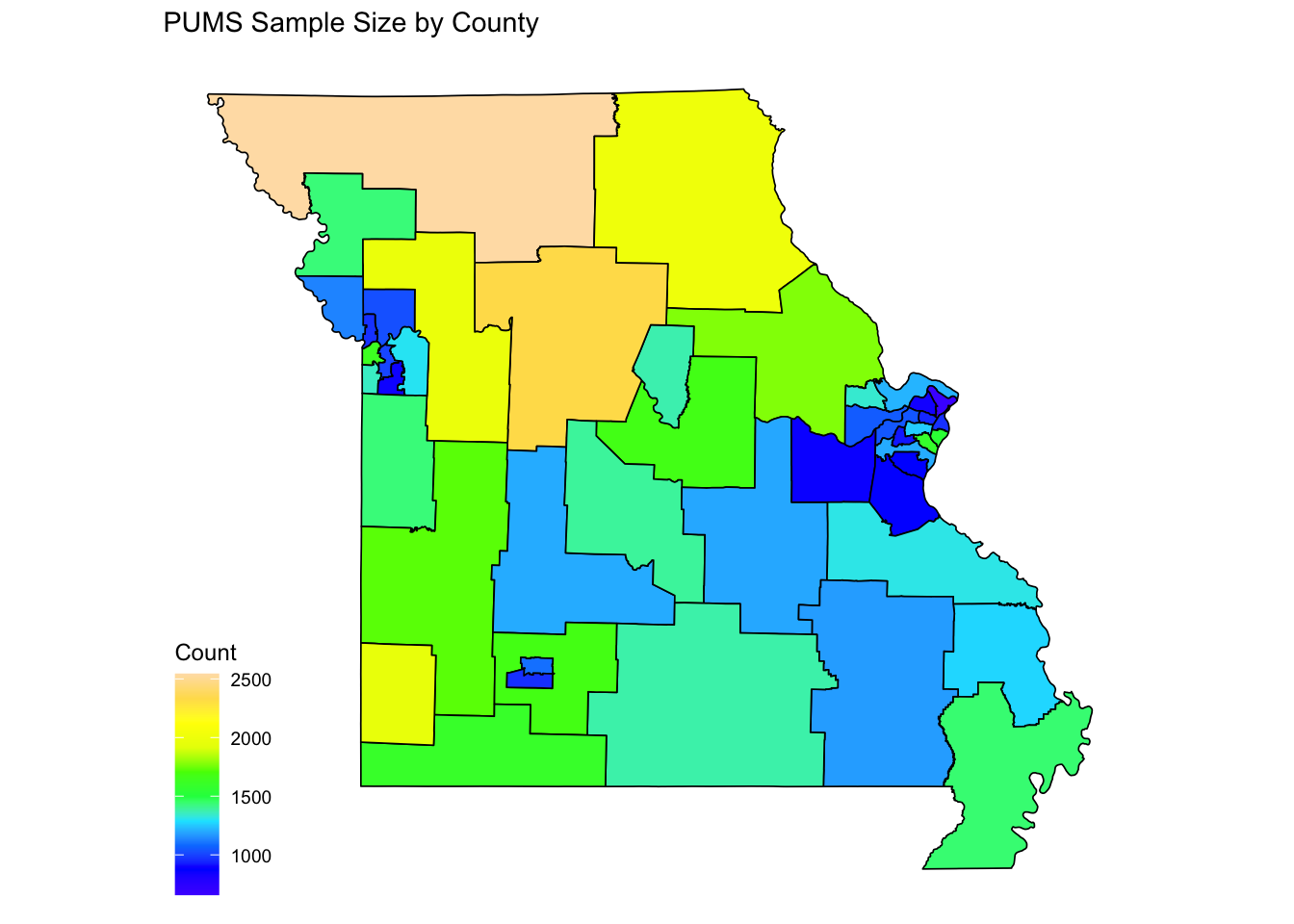# Plotting PUMAs in R

If you work with Census Bureau data, you are probably familiar with Public Use Microdata Samples (PUMS) from the American Community Survey. These are individual records (either person or household) that allow data users more customized analysis than would be available with the ACS tabulated data products. In order to preserve confidentiality, the geographic information in this data is limited to the Public Use Microdata Area (PUMA). This is a custom geographic area that nests within state and is defined in such a way that each PUMA contains at lease 100,000 people.

When working with PUMS data, it is often desirable to create a map as a visualization tool, which is the main goal of this post. To begin, the `pumas()` function in the `tigris` package can be used to easily download PUMA shapefiles. I will demonstrate for the state of Missouri.

``````library(tigris)
pumasMO <- pumas("MO", cb=T)``````

The argument `cb=T` is used to download a general shape file rather than a high detail one.

``class(pumasMO)``
``````##  "SpatialPolygonsDataFrame"
## attr(,"package")
##  "sp"``````

Note that this object has the class `SpatialPolygonsDataFrame`. We use the `fortify()` function in the `ggplot2` package to convert this into a nice tidy dataframe.

``````library(ggplot2)
pumasMO <- fortify(pumasMO, region='PUMACE10')``````

Next, we read in the PUMS data that we are working with.

``````library(readr)

For simplicity, I am just going to count the number of sampled individuals within each PUMA, which I will later plot.

``````library(dplyr)
pumsMO <- pumsMO %>% group_by(PUMA) %>% summarize(Count=n())
str(pumsMO)``````
``````## tibble [47 × 2] (S3: tbl_df/tbl/data.frame)
##  \$ PUMA : chr [1:47] "00100" "00200" "00300" "00400" ...
##  \$ Count: int [1:47] 2543 1432 2015 1775 1674 1372 2331 1977 1026 964 ...``````

The final step is to join the PUMS data that we want to plot with the tidy PUMA data that we created earlier.

``````plotDF <- pumasMO %>% left_join(pumsMO, by=c("id"="PUMA"))
str(plotDF)``````
``````## 'data.frame':    13680 obs. of  8 variables:
##  \$ long : num  -95.8 -95.8 -95.8 -95.7 -95.7 ...
##  \$ lat  : num  40.6 40.6 40.6 40.6 40.6 ...
##  \$ order: int  1 2 3 4 5 6 7 8 9 10 ...
##  \$ hole : logi  FALSE FALSE FALSE FALSE FALSE FALSE ...
##  \$ piece: Factor w/ 2 levels "1","2": 1 1 1 1 1 1 1 1 1 1 ...
##  \$ id   : chr  "00100" "00100" "00100" "00100" ...
##  \$ group: Factor w/ 48 levels "00100.1","00200.1",..: 1 1 1 1 1 1 1 1 1 1 ...
##  \$ Count: int  2543 2543 2543 2543 2543 2543 2543 2543 2543 2543 ...``````

Now we can make a map that shows the areal data we are interested in.

``````library(ggthemes)
ggplot(plotDF, aes(x=long, y=lat, group=group))+
geom_polygon(color='black', size=0.3, aes(fill=Count))+
coord_map()+
theme_map()+